# An acid is titrated with NaOH. The following beakers are illustrations of the contents of the beaker at various times during the titration. These are presented out of order. Note: Counter-ions and water molecules have been omitted from the illustrations for clarity. ( a ) ( b ) ( c ) ( d ) ( e ) a. Is the acid a weak or strong acid? How can you tell? b. Arrange the beakers in order of what the contents would look like as the titration progresses. c. For which beaker would pH = p K a ? Explain your answer. d. Which beaker represents the equivalence point of the titration? Explain your answer. e. For which beaker would the K a value for the acid not be necessary to determine the pH? Explain your answer.### Chemistry: An Atoms First Approach

2nd Edition
Steven S. Zumdahl + 1 other
Publisher: Cengage Learning
ISBN: 9781305079243

#### Solutions

Chapter
Section### Chemistry: An Atoms First Approach

2nd Edition
Steven S. Zumdahl + 1 other
Publisher: Cengage Learning
ISBN: 9781305079243
Chapter 14, Problem 13Q
Textbook Problem
31 views

## An acid is titrated with NaOH. The following beakers are illustrations of the contents of the beaker at various times during the titration. These are presented out of order. Note: Counter-ions and water molecules have been omitted from the illustrations for clarity.(a)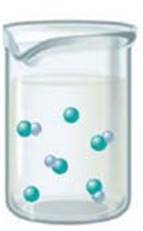(b)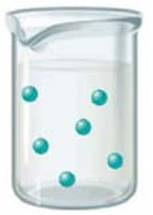(c)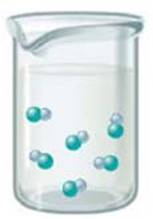(d)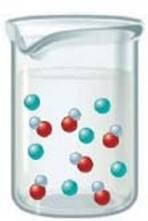(e)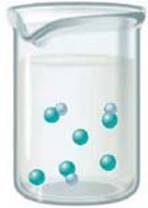a. Is the acid a weak or strong acid? How can you tell?b. Arrange the beakers in order of what the contents would look like as the titration progresses.c. For which beaker would pH = pKa? Explain your answer.d. Which beaker represents the equivalence point of the titration? Explain your answer.e. For which beaker would the Ka value for the acid not be necessary to determine the pH? Explain your answer.

(a)

Interpretation Introduction

Interpretation:

The illustrations of the contents of a beaker corresponding to the titration of an acid with NaOH are given. The questions related to this are to be answered.

Concept introduction:

The point, at which there is just enough OH to neutralize the initial amount of acid, is known as the equivalence point.

To determine: If the given acid is a weak or a strong acid.

### Explanation of Solution

Explanation

A weak acid does not completely dissociate into its ions in the solution. It is present in its undissociated form...

(b)

Interpretation Introduction

Interpretation:

The illustrations of the contents of a beaker corresponding to the titration of an acid with NaOH are given. The questions related to this are to be answered.

Concept introduction:

The point, at which there is just enough OH to neutralize the initial amount of acid, is known as the equivalence point.

To determine: The correct arrangement of the beakers in the order in which the given titration would occur.

(c)

Interpretation Introduction

Interpretation:

The illustrations of the contents of a beaker corresponding to the titration of an acid with NaOH are given. The questions related to this are to be answered.

Concept introduction:

The point, at which there is just enough OH to neutralize the initial amount of acid, is known as the equivalence point.

(d)

Interpretation Introduction

Interpretation:

The illustrations of the contents of a beaker corresponding to the titration of an acid with NaOH are given. The questions related to this are to be answered.

Concept introduction:

The point, at which there is just enough OH to neutralize the initial amount of acid, is known as the equivalence point.

To determine: The beaker that represents the equivalence point in the titration.

(e)

Interpretation Introduction

Interpretation:

The illustrations of the contents of a beaker corresponding to the titration of an acid with NaOH are given. The questions related to this are to be answered.

Concept introduction:

The point, at which there is just enough OH to neutralize the initial amount of acid, is known as the equivalence point.

### Still sussing out bartleby?

Check out a sample textbook solution.

See a sample solution

#### The Solution to Your Study Problems

Bartleby provides explanations to thousands of textbook problems written by our experts, many with advanced degrees!

Get Started